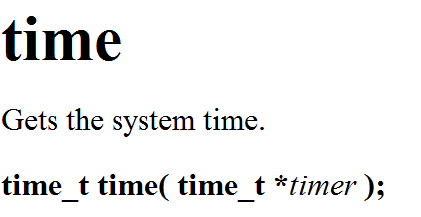WHCSRL 技术网

# 请回答c语言-分支和循环结构【入门】

• if
• switch

• while
• for
• do while

goto语句

## 1.五种语句

1. 表达式语句
2. 函数调用语句
3. 控制语句
4. 复合语句
5. 空语句

C语言有九种控制语句，可分成以下三类：

1. 条件判断语句也叫分支语句：if语句、switch语句；
2. 循环执行语句：do while语句、while语句、for语句；
3. 转向语句：break语句、goto语句、continue语句、return语句。

## 2. 分支语句（选择结构）

### 2.1 if 语句

#### 2.1.1 if 语句语法结构

``````//语法结构：
//单
if(表达式)
语句;
//双
if(表达式)
语句1;
else
语句2;
//多分支
if(表达式1)
语句1;
else if(表达式2)
语句2;
else
语句3;
```12345678910111213141516```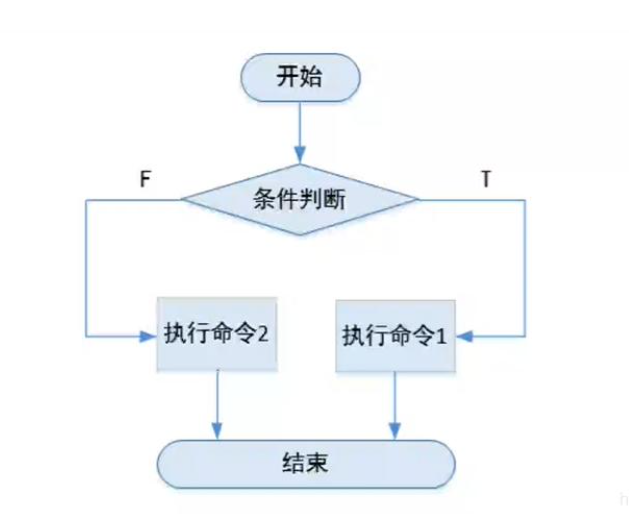if为真则执行语句，否则不执行该语句

0表示假，非0表示真

#### 2.1.1 if语句书写

for example:

``````#include <stdio.h>

int main()
{
int level = 0;
scanf("%%d", &level);
if (level < 16)
{
printf("小火龙
");
}
else if (level >= 16 && level < 36)
{
printf("火恐龙
");
}
else if(level>=36&&level<=100)
{
printf("喷火龙
");
}
else//悬垂else，最后一个else可以不用写条件
{
printf("不可能");
}
```12345678910111213141516171819202122```

``````if(16<=level<36)
```1```

#### 2.1.2 if 语句代码风格

``````//代码1
if (condition) {
return x;
}
return y;
//代码2
if (condition)
{
return x;
}
else
{
return y;
}
//代码3
int num = 1;
if (num == 5)
{
printf("hehe
");
}
//代码4
int num = 1;
if (5 == num)
{
printf("hehe
");
}
```1234567891011121314151617181920212223242526```

``````//打印1-100之间的奇数
int main()
{
int i = 0;
for(i=1;i<100;i++)
{
if (i %% 2 != 0)
printf("%%d ", i);
}
return 0;
}
```1234567891011```

### 2.2 switch语句

switch语句也是一种分支语句。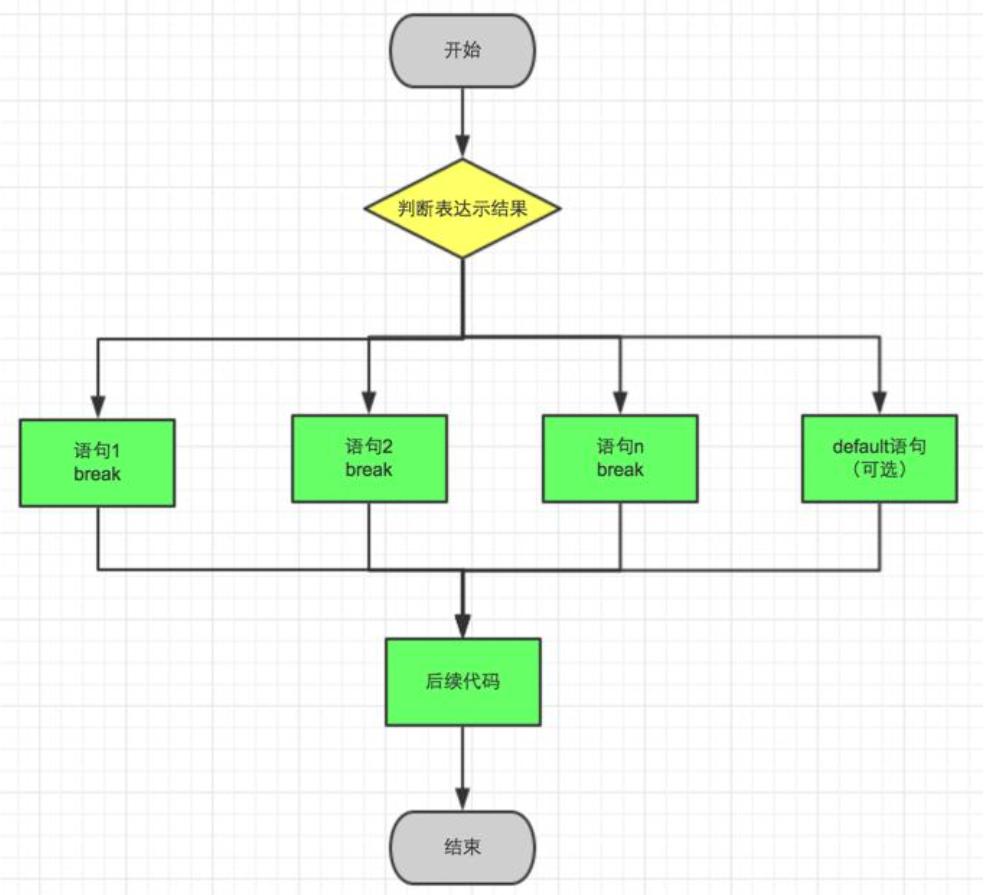#### 2.2.1 switch的语句结构

``````switch(整型表达式)
{
语句项；
}
> 而语句项是什么呢？
//是一些case语句：
//如下：
case 整形常量表达式:
语句;
```123456789```

#### 2.2.2 break in the switch

``````int main()
{
int input = 0;
scanf("%%d", &input);
switch (input)
{
case 1:
printf("LEBRON
");
break;
case 2:
printf("CURRY
");
break;
case 3:
printf("KD
");
break;
case 4:
printf("HARDON
");
break;
case 5:
printf("PG
");
break;
case 6:
printf("GIANNIS
");
break;
case 7:
printf("077
");
break;
}
}
```1234567891011121314151617181920212223242526272829```

``````int main()
{
int input = 0;
scanf("%%d", &input);
switch (input)
{
case 1:
case 2:
case 3:
case 4:
case 5:
printf("LEBRON
");
break;
case 6:
printf("GIANNIS
");
break;
case 7:
printf("077
");
break;
}
}
```123456789101112131415161718192021```

break语句 的实际效果是把语句列表划分为不同的分支部分

## 3. 循环语句

• while
• for
• do while

### 3.1 while循环

C语言中给我们引入了： while 语句，可以实现循环。

``````//while 语法结构
while(表达式)

```123```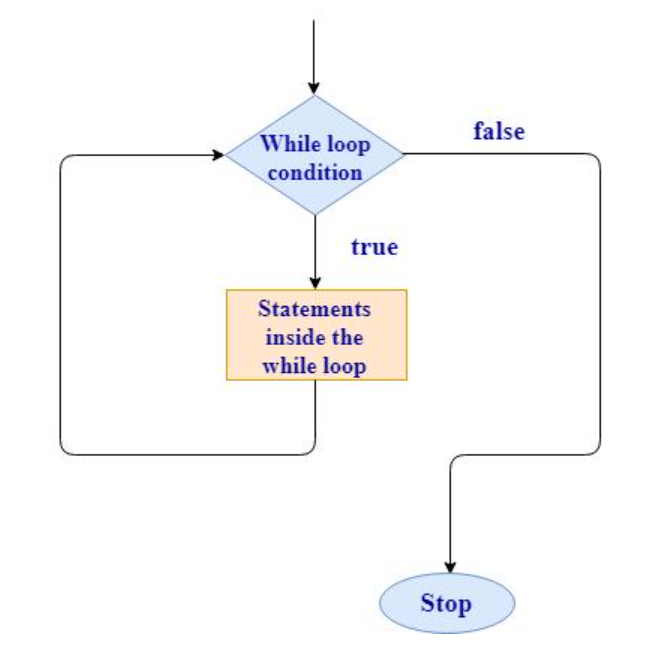#### 3.1.1 while语句书写

``````int main()
{
int i = 1;
while (i <= 100)
{
printf("%%d ", i);
i += 2;
}
return 0;
}
```12345678910```

#### 3.1.2 break and continue in while

##### 3.1.2.1 break

``````#include <stdio.h>
int main()
{
int i = 1;
while(i<=10)
{
if(i == 5)
break;
printf("%%d ", i);
i = i+1;
}
return 0;
}
```12345678910111213```

break在while循环中的作用：

##### 3.1.2.2 continue

continue的作用是跳过本次循环continue后面的代码

``````#include <stdio.h>
int main()
{
int i = 1;
while (i <= 10)
{
if (i == 5)
continue;
printf("%%d ", i);
i = i + 1;
}
return 0;
}
```12345678910111213```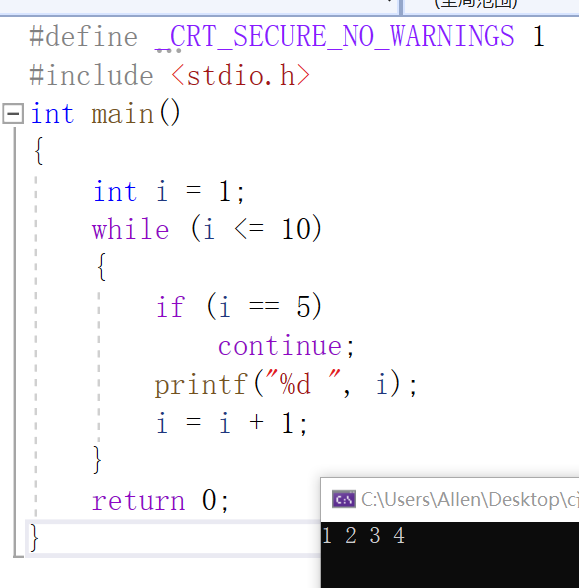continue在while循环中的作用就是：
continue是用于终止本次循环的，也就是本次循环中continue后边的代码不会再执行，而是直接跳转到while语句的判断部分。进行下一次循环的入口判断。

##### 3.1.3 while循环例子

``````#include <stdio.h>

int main()
{
int ch = 0;
while ((ch = getchar()) != EOF)
putchar(ch);
return 0;
}
```123456789```

getchar函数是接受输入流的标准输入函数，当读取失败后会返回EOF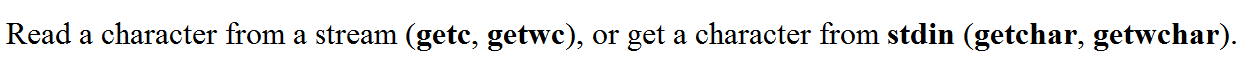getchar和scanf类似，不同的是getchar只能用来打印标准输入（stdin）中的字符，而scanf可以打印浮点型和整型

putchar函数类似于printf，用来向标准输出（stdout）打印字符数据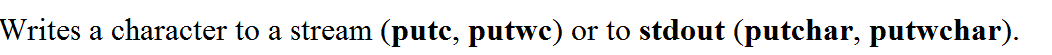### 3.2 for 循环

#### 3.2.1 语法

``````for(表达式1; 表达式2; 表达式3)

```12```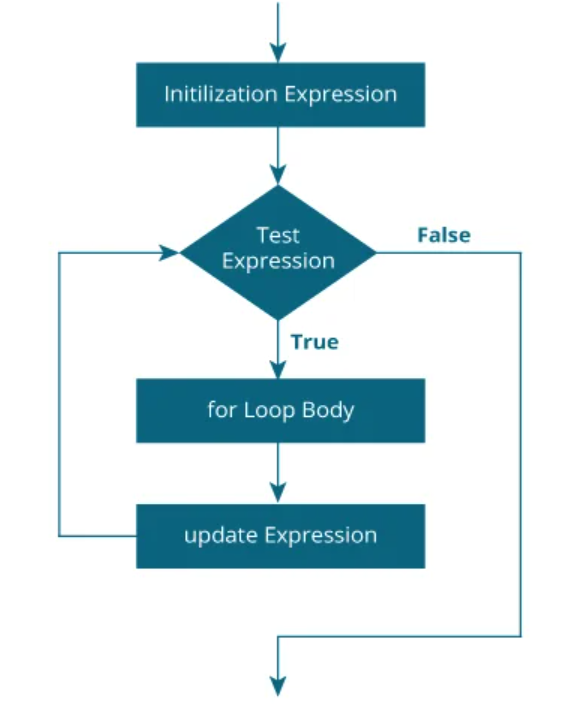#### 3.2.2 for语言的书写

``````int main()
{
for (int i = 1; i < 100; i+=2)
{
printf("%%d ", i);
}
return 0;
}
```12345678```

#### 3.2.3 break and continue

##### 3.2.3.1 break in the for

break是直接结束该循环的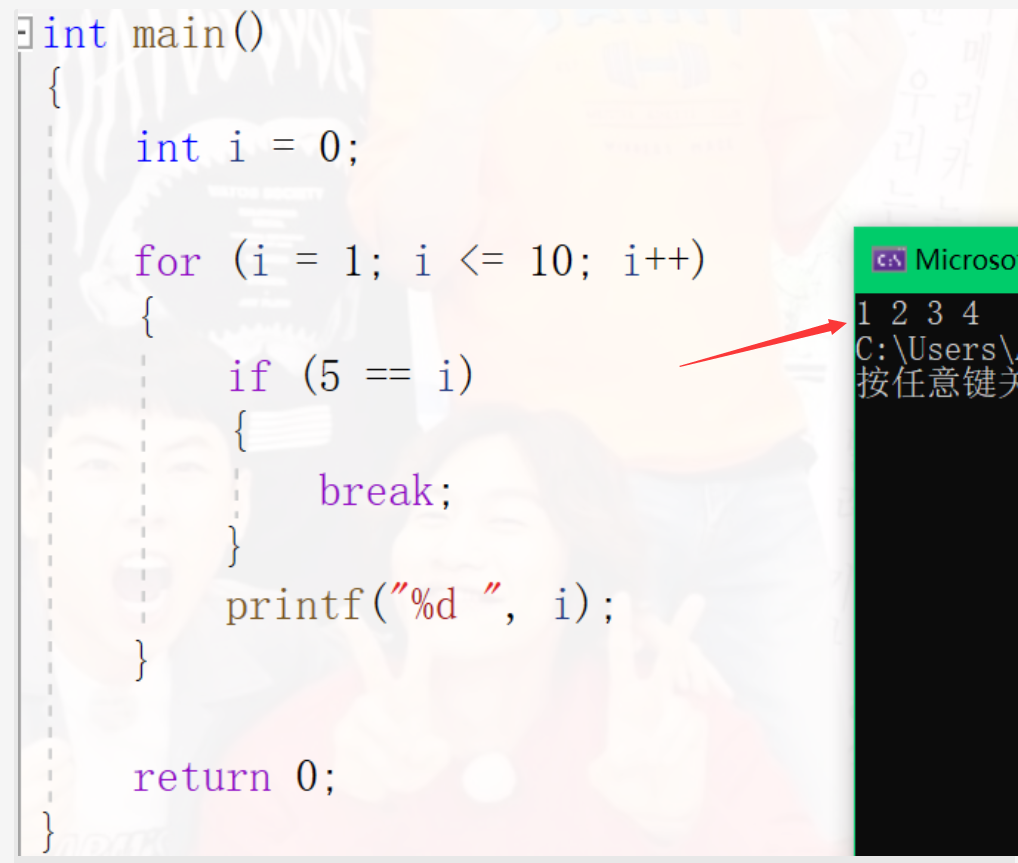##### 3.2.3.2 continue in the for

continue只会跳过这次循环剩下的过程在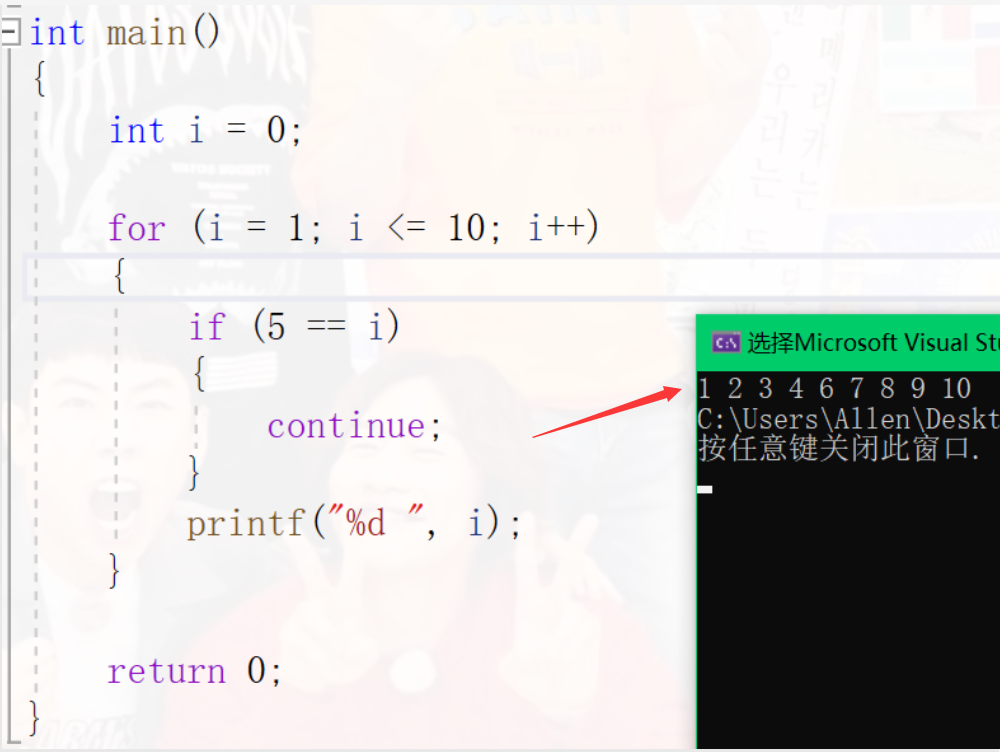#### 3.2.4 for语句的循环控制变量

1. 最好不要在for 循环体内修改循环变量，防止 for 循环失去控制。
2. for语句的循环控制变量的取值建议采用“前闭后开区间”写法。
``````//前闭后开的写法
int i = 0;
for(i=0; i<10; i++)
{
...;
}
```123456```

#### 3.2.5 for循环的其他写法

1.for循环中的初始化部分，判断部分，调整部分是可以省略的

``````for(;;)
{
printf("我是for循环
");
}
```1234```

``````int i = 0;
int j = 0;
//这里打印多少个9012?
for (int i=0; i < 10; i++)
{
for (; j < 10; j++)
{
printf("9012
");
}
}
```12345678910```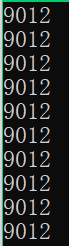for循环是平时使用很多的循环

### 3.3 do while 循环

do while循环至少执行一次，可是这个语句使用的场景有限，所以不是经常使用。

#### 3.3.1 do while语句的语法

``````do
循环语句;
while(表达式);
```123```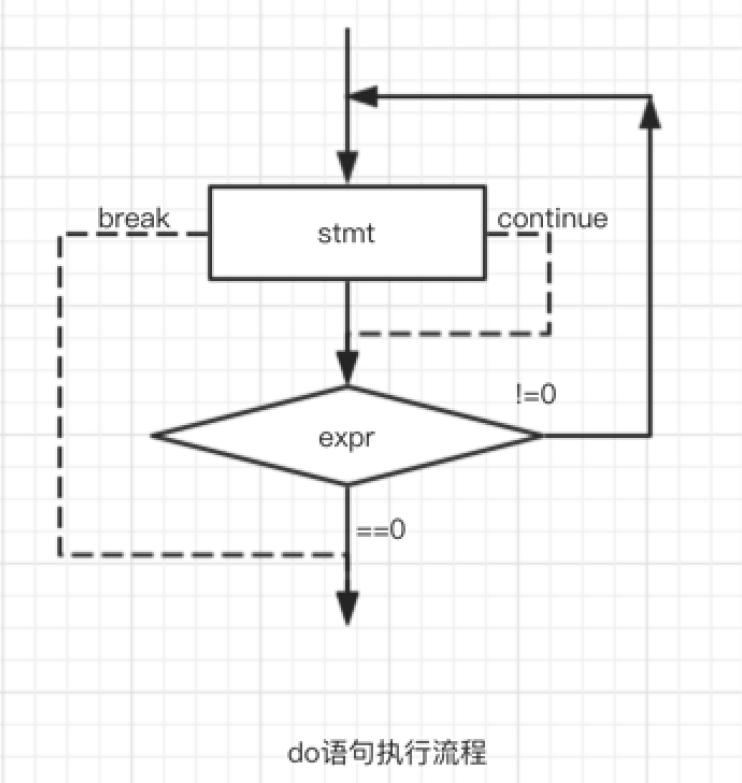#### 3.3.2 do while 语句的书写

``````int main()
{
int i = 10;
do
{
printf("%%d
", i);
} while (i < 10);
return 0;
}
```123456789```

#### 3.3.3 do while循环中的break和continue

##### 3.3.3.1 break in the do while

``````int main()
{
int i = 10;
do
{
if (5 == i)
break;
printf("%%d
", i);
} while (i < 10);
return 0;
}
```1234567891011```
##### 3.3.3.2 continue in the do while

``````int main()
{
int i = 10;
do
{
if (5 == i)
continue;
printf("%%d
", i);
} while (i < 10);
return 0;
}
```1234567891011```

## 4.练习提高

1. 计算 n的阶乘
``````int main()
{
int n = 0;
scanf("%%d", &n);//5
int i = 0;
int ret = 1;
for (i = 1; i <= n; i++)
{
ret = ret * i;
}
printf("%%d
", ret);
return 0;
}
```12345678910111213```
1. 计算 1!+2!+3!+……+10!
``````int main()
{
int n = 0;
int i = 0;
int ret = 1;
int sum = 0;
for (n = 1; n <= 10; n++)
{
ret = 1;//再从1开始乘，这步不能忘
for (i = 1; i <= n; i++)
{
ret = ret * i;
}
sum += ret;
}
printf("%%d
", sum);
return 0;
}
```123456789101112131415161718```
1. 打印99乘法表
``````int main()
{
int i = 0;
int j = 0;
for (i = 1; i <= 9; i++)
{
for (j = 1; j <= i; j++)
{
printf("%%d*%%d=%%d",i,j, i * j);
printf("	");
}
printf("
");
}
}

```123456789101112131415```

1. 在一个有序数组中查找具体的某个数字n。（二分查找法）
``````int main()
{
int arr[] = { 1,2,3,4,5,6,7,8,9,10 };
int sz = sizeof(arr) / sizeof(arr);//取得数组长度写法
int num = 0;
scanf("%%d",&num);
int left = 0;
int right = sz - 1;
while (left<=right)
{
int mid = (left + right) / 2;
if (arr[mid] < num)
{
left = mid + 1;
}
else if (arr[mid] > num)
{
right = mid - 1;
}
else
{
printf("找到了，下标是:%%d
", mid);
break;
}
}
if (left > right)
{
printf("找不到
");
}
return 0;
}
```12345678910111213141516171819202122232425262728293031```
1. 编写代码，演示多个字符从两端移动，向中间汇聚。

``````int main()
{
char arr1[] = "I can do all things";
char arr2[] = "###################";
int left = 0;
int right = strlen(arr1) - 1;
printf("%%s
", arr2);
//while循环实现
while (left <= right)
{
Sleep(500);
arr2[left] = arr1[left];
arr2[right] = arr1[right];
left++;
right--;
printf("%%s
", arr2);
}
//for循环实现
for (left = 0, right = strlen(arr1) - 1;
left <= right;
left++, right--)
{
Sleep(500);
arr2[left] = arr1[left];
arr2[right] = arr1[right];
printf("%%s
", arr2);
}
return 0;
}
```1234567891011121314151617181920212223242526272829```
1. 编写一个密码

``````#include<stdio.h>
#include<string.h>
int main()
{
int i = 0;
//这里我们假设密码是123456
for (i = 0; i < 3; i++)
{
printf("请输入密码：");
{
printf("登陆成功
");
break;
}
else
{
printf("密码错误
");
}
}
if(3==i)
{
printf("输错三次密码退出程序
");
}
return 0;
}
```123456789101112131415161718192021222324252627```

strcmp 在MSDN上是这么写的：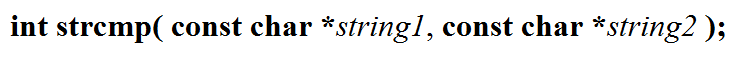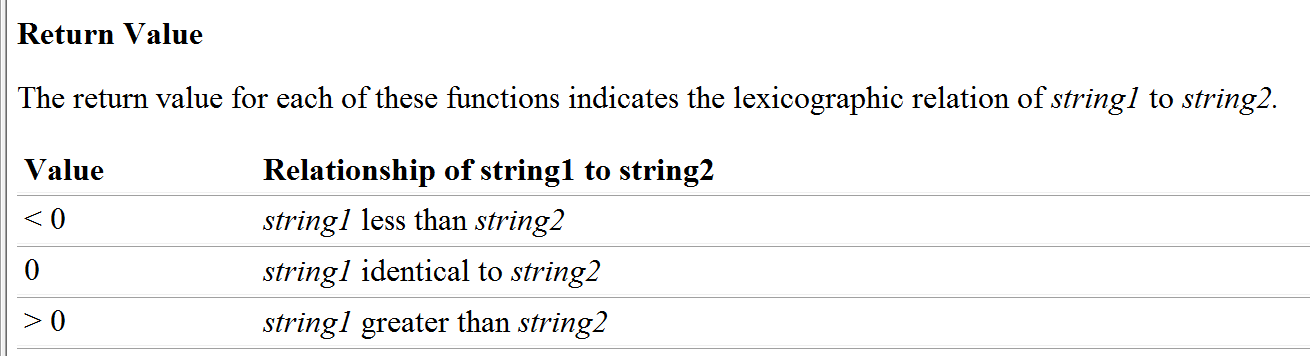1. 猜数字游戏（电脑随机生成1-100）的数字

``````#include<stdlib.h>
#include<stdio.h>
#include<time.h>
{
printf("***************************
");
printf("*****    1. play     ******
");
printf("*****    0. exit     ******
");
printf("***************************
");
}
void game()
{
int guess = 0;
//猜数字游戏的过程
//播撒随机数种子
int r=rand()%%100+1;
//猜数字

while(1)
{
printf("猜数字：
");
scanf("%%d",&guess);
if(guess<r)
{
printf("猜小了
");
}
else if(guess>r)
{
printf("猜大了
");
}
else
{
printf("恭喜你，猜对了!
");
break;
}
}
}
int main()
{
int input=0;
srand((unsigned int)time(NULL));//放在主函数里面只要一次就够了

do
{
//打印菜单
printf("请选择");
scanf("%%d",&input);
switch(input)
{
case 1:
game();
break;
case 0:
printf("退出游戏
");
break;
default:
printf("选择错误
");
break;
}
}while(input);
return 0;
}
```123456789101112131415161718192021222324252627282930313233343536373839404142434445464748495051525354555657585960616263```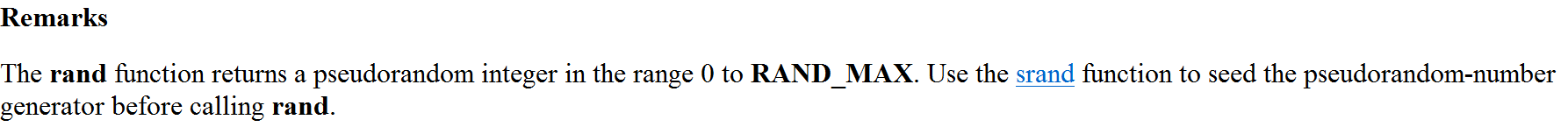srand需要用时间戳来随机播撒种子，关于时间戳的概念可以自行搜索,利用time函数，如下：